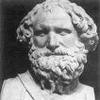#### You may also likeA 1 metre cube has one face on the ground and one face against a wall. A 4 metre ladder leans against the wall and just touches the cube. How high is the top of the ladder above the ground?### Growing

Which is larger: (a) 1.000001^{1000000} or 2? (b) 100^{300} or 300! (i.e.factorial 300)### Archimedes and Numerical Roots

The problem is how did Archimedes calculate the lengths of the sides of the polygons which needed him to be able to calculate square roots?

# Back Fitter

### Why do this problem?

This problem offers an opportunity to reflect on the very important concept of fitting a curve to experimental data. Along the way, students will utilise their skills of transforming graphs in order to find a close fit, and consider ways of deciding how close their fit is. The problem is marked as challenge level 1 as it is a straightforward task to begin, but to find a complete solution for all 10 graphs is rather more challenging!

### Possible approach

Although this problem stands alone, it could also be done as a follow-up to work on transformations of graphs based on the problem Parabolic Patterns.

Part of the challenge of this problem is to identify which graphs are easiest to fit, as they are not presented in any particular order. One approach is to start by displaying the graphs and discussing as a class or in pairs which have recognisable shapes, such as straight lines, quadratics, trig graphs and exponential graphs.

If students haven't met graphs such as $y=a^x$ and $y=a^{-x}$ it might be fruitful to give them some time to experiment with graphical calculators to see what these graphs look like for different values of the constant $a$.

Once students have some preliminary ideas about graphs which might fit, small groups could start to work on the spreadsheet, entering a possible equation and seeing how closely it matches the given data, then using their knowledge of transformations of graphs to tweak their equation to get a closer match. Alternatively, they could experiment with graphical calculators to find graphs with the right basic shape and then enter them into one copy of the spreadsheet displayed at the front of the class.

Ideally, different groups will come up with slightly different suggestions for functions, and this can stimulate discussion about how to decide which function most closely matches the data.

### Key questions

What clues can we find from the axes and the points given to help us to guess a likely function?

How can we modify our guess once we've seen how closely it fits?
Does joining the points in order of increasing time help?
How do we decide when the fit is close enough?

### Possible support

Graphs 1, 3 and 5 are the most straightforward functions to fit, so this is a good place to start.

### Possible extension

Students could investigate and discuss the benefits of a least squares method of determining how close the fit is.